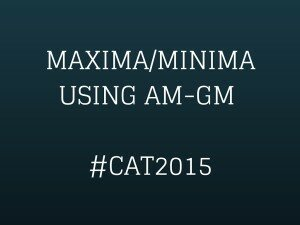Select PageWe will look at finding the maxima/minima using the AM-GM inequality in this article. This is part of the article series we are doing in the run up to CAT 2015. Here are the other articles in this series: 75 Days to CAT.

Concept

If we have 2 numbers ‘a’ and ‘b’,

the arithmetic mean AM = (a+b)/2

whereas the geometric mean GM = √(ab)

Similarly, for 3 numbers, the arithmetic mean would be average of the three numbers whereas the geometric mean would be cube root of the product of the three numbers.

In general, the AM-GM inequality states that for any positive numbers,

AM ≥ GM

Hence, for 2 numbers, (a+b)/2 ≥ √(ab)

We can use this in questions to find the maximum value of the product of numbers if the sum is given. Alternatively, we can use this to find the minimum value of the sum of numbers if the product is given.

Let’s see this with the help of examples.

Examples

1. The sum of two positive numbers is 14. What is the maximum value of their product?

Let the two numbers be a and b. We know that a+b = 14.

Hence, AM = (a+b)/2 = 14/2 = 7

Since AM ≥ GM, (a+b)/2 ≥ √(ab)

=>  √(ab) ≤ 7

=> ab ≤ 49

Hence, the maximum value of their product is 49.

1. The product of two positive numbers is 100. What is the minimum value of their sum?

Let the two numbers be a and b. We know that ab = 100.

Hence, GM =  √(ab) = 10.

Since AM ≥ GM, (a+b)/2 ≥ √(ab)

=>  (a+b)/2 ≥ 10

=>  (a+b) ≥ 20.

1. The sum of three positive numbers is 30. What is the maximum value of their product?

Let the two numbers be a, b and c. We know that a+b+c = 30.

Logically, from the 2 examples above, for the product to be maximum, the three numbers have to be equal. Hence a=b=c=10.

Hence, the maximum value of the product will be abc = 10*10*10 = 1000.

Let’s take a slightly trickier question.

1. The sum of three positive numbers a, b and c is 30. What is the maximum value of ab2c2?

We know that a+b+c = 30.

We know that ab2c2 will come in the GP side of the inequality AM ≥ GM. However, to get this inequality in the GM side, the AM side should have 1 a term, 2 b terms and 2 c terms. Only will then the product of the terms give the expression ab2c2.

Hence, we need to manipulate the sum of the three numbers so as to have 1 a term, 2 b terms and 2 c terms. We can do this by writing the equation as a + b/2 + b/2 + c/2 + c/2 = 30.

Hence, the term ab2c2 will be maximized when a = b/2 = b/2 = c/2 = c/2.

Since, a+b+c = 30

=>  a+2a+2a=30

=>  5a=30

=>  a=6

=>  b=c=12

Hence, the maximum value of ab2c2 is 6*122*122= 124,416.

error: Content is protected !!